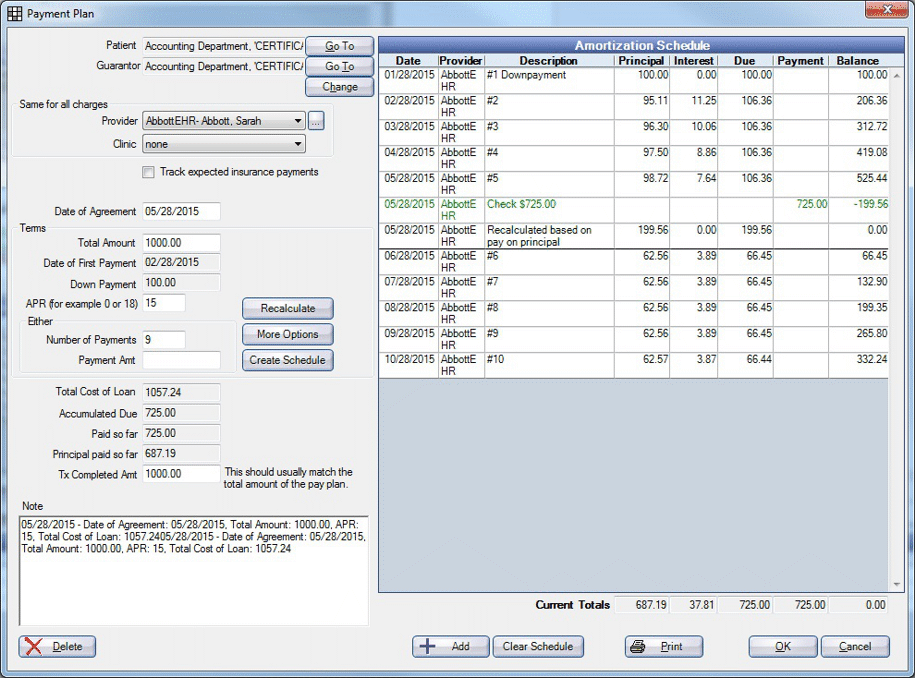Payment Plan Unit Test Screens

These screens represent the different Payment Plan Unit Tests scenarios.

Down equals total amount.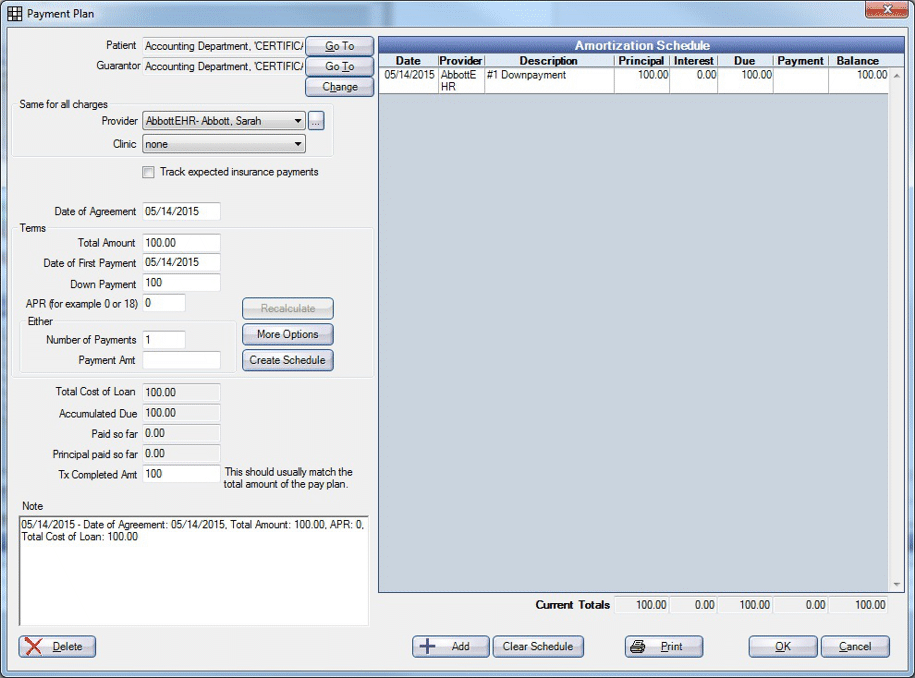Number of payments simple.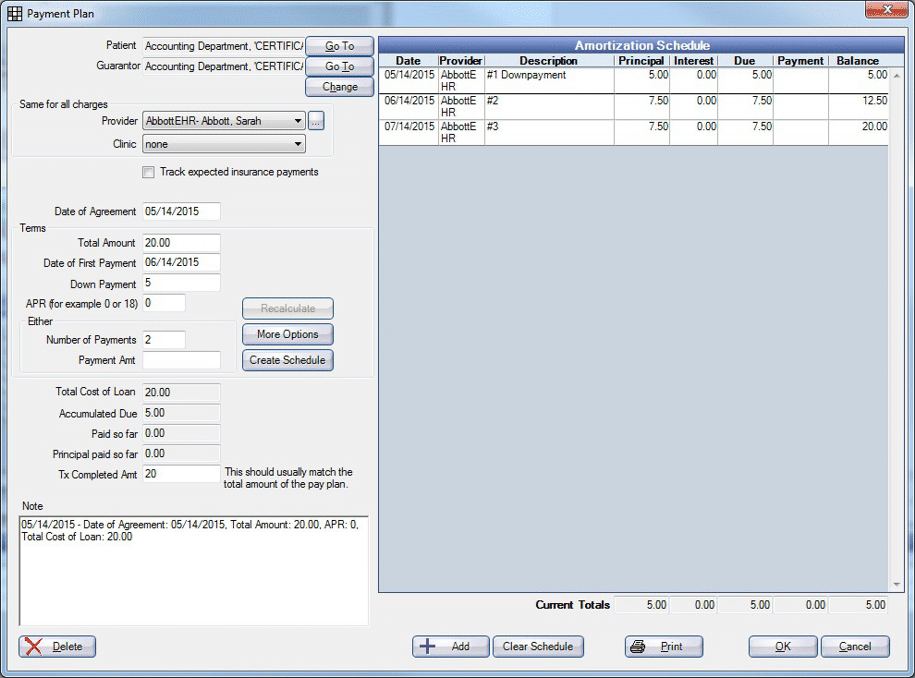Term example.Term example.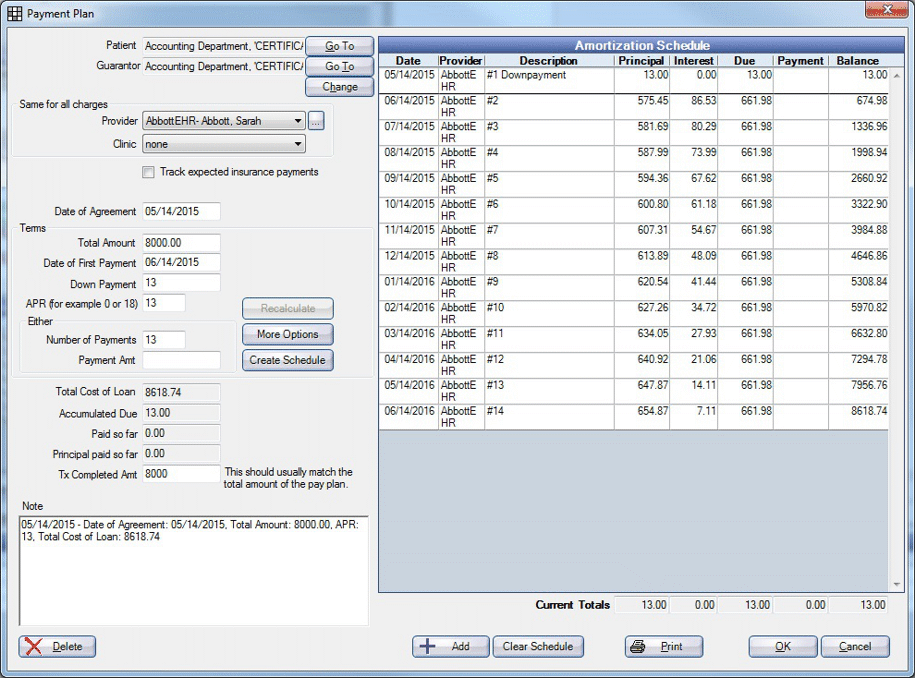Real world example.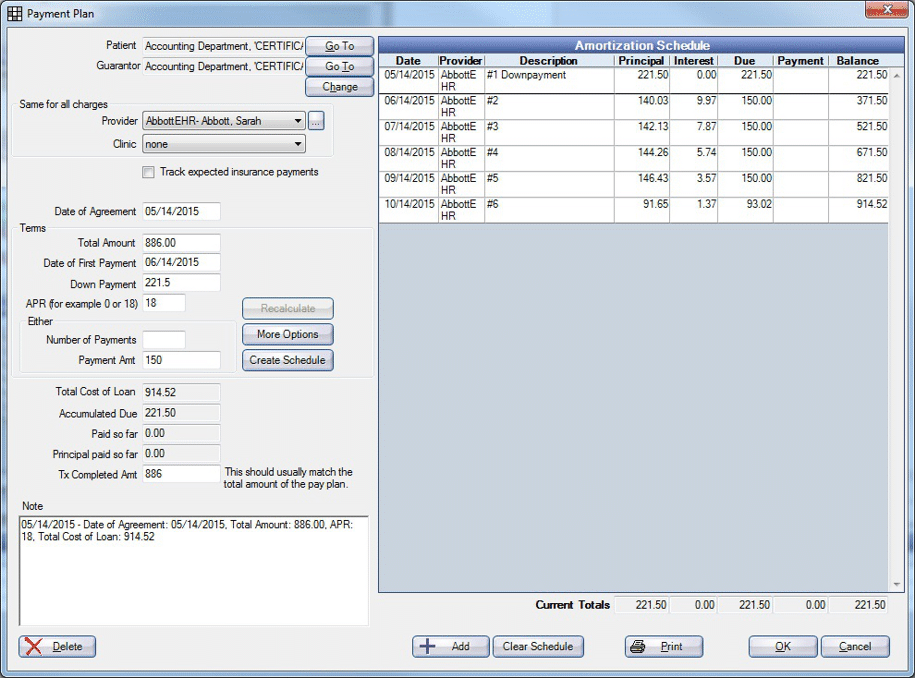Payment amount example.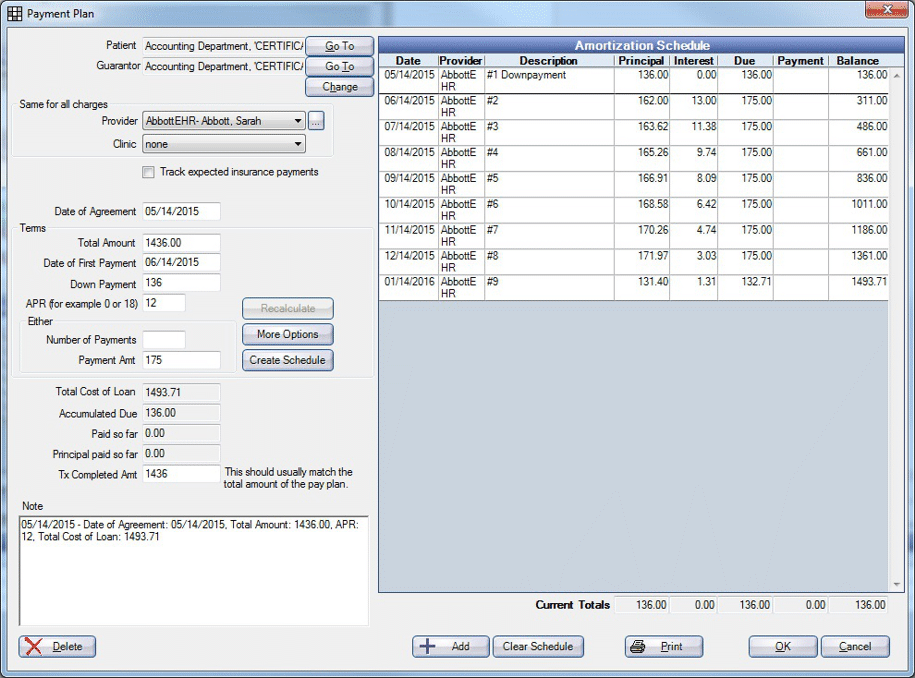Term plan with no interest and different final payment amount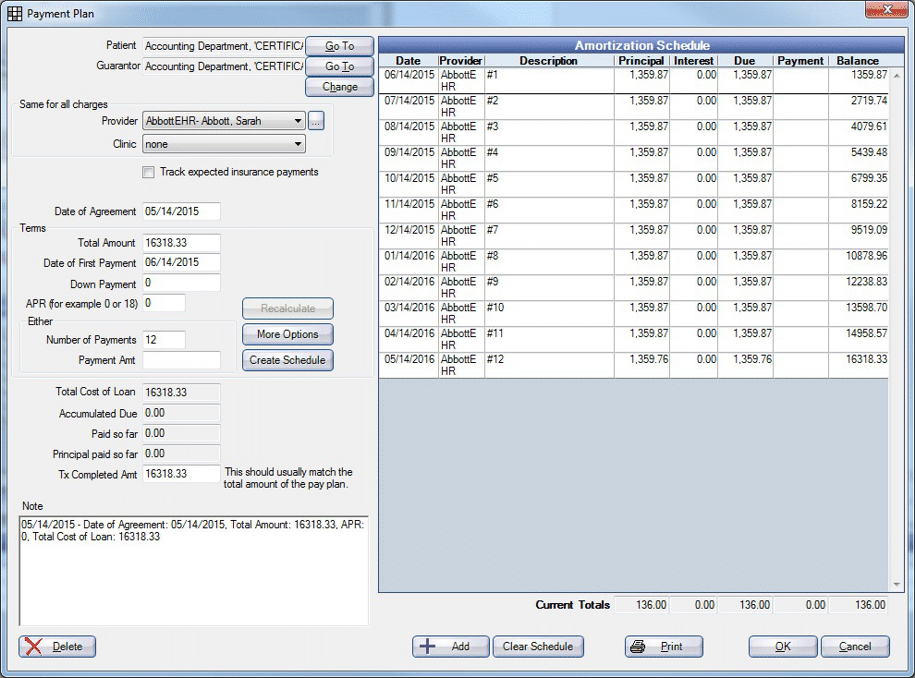## Recalculations

1. Balance Due on Account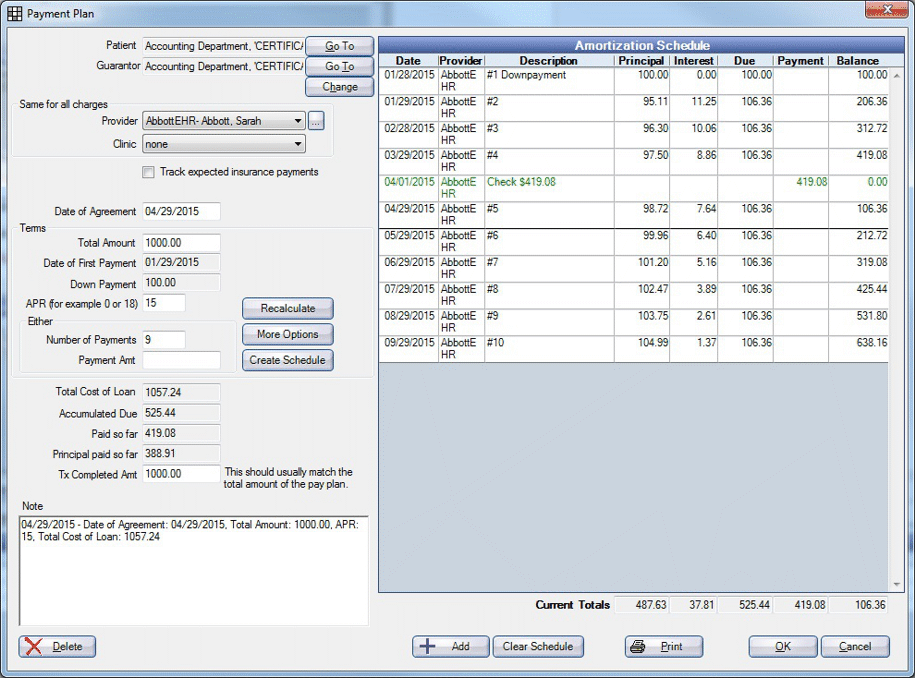1a. Balance Due: If you select Prepay and Recalculate Interest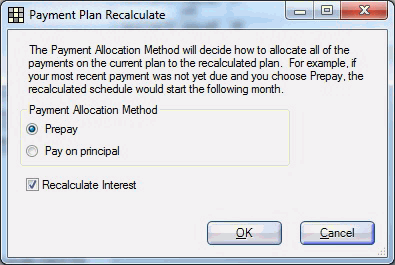1b. Balance Due: If you select Prepay and do not Recalculate Interest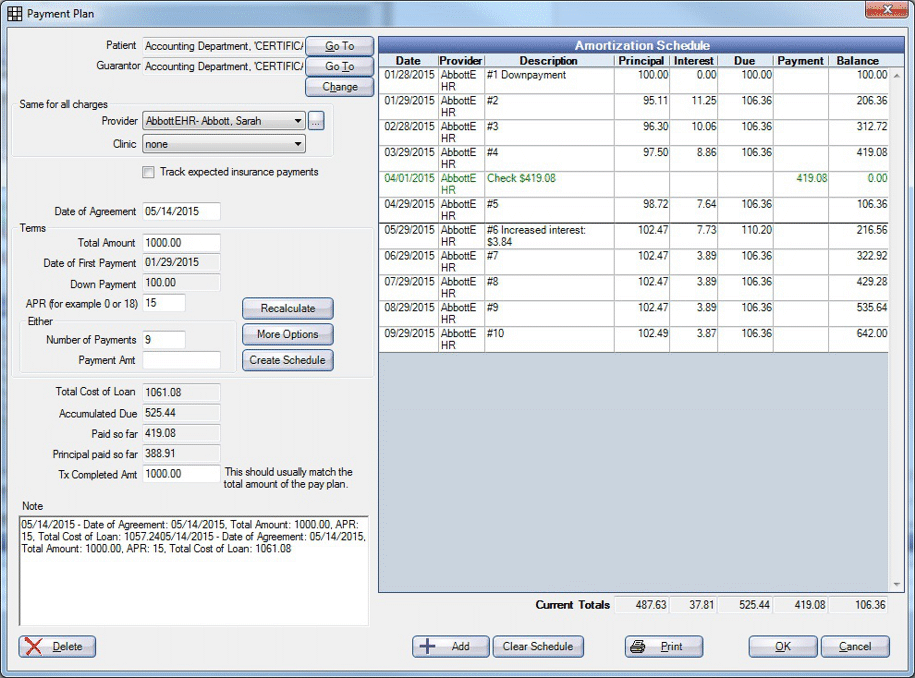1c. Balance Due: If you select Pay on Principal and Recalculate Interest1d. Balance Due: If you select Pay on Principal and do not Recalculate Interest.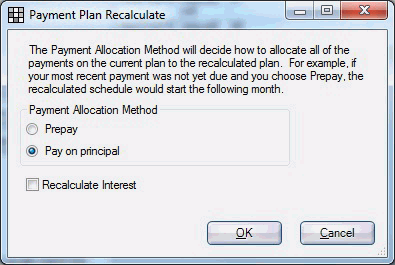2. Overpaid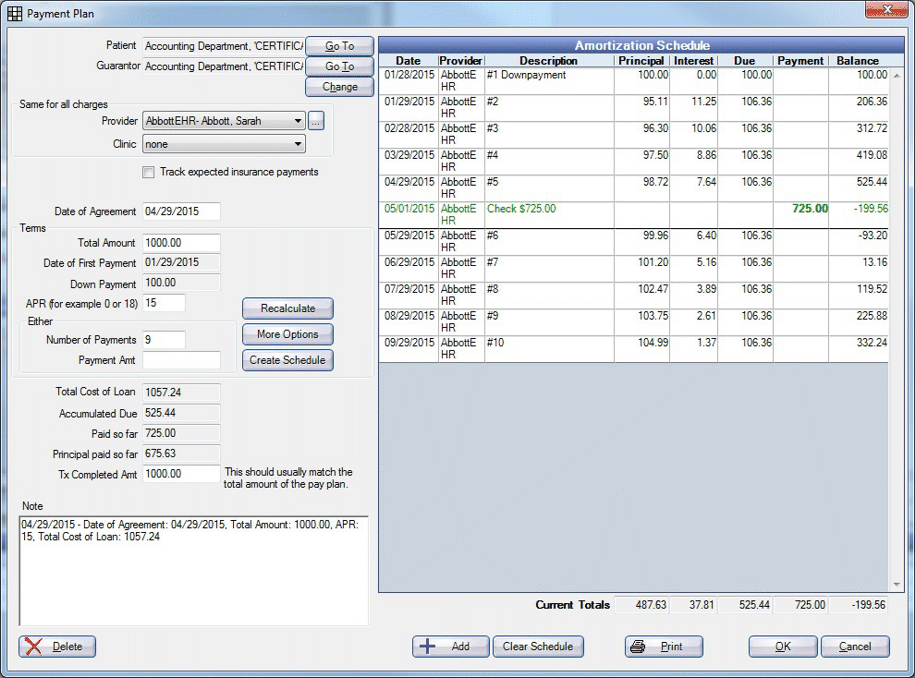2a. Overpaid: If you select Prepay and Recalculate Interest2b. Overpaid: If you select Prepay and do not Recalculate Interest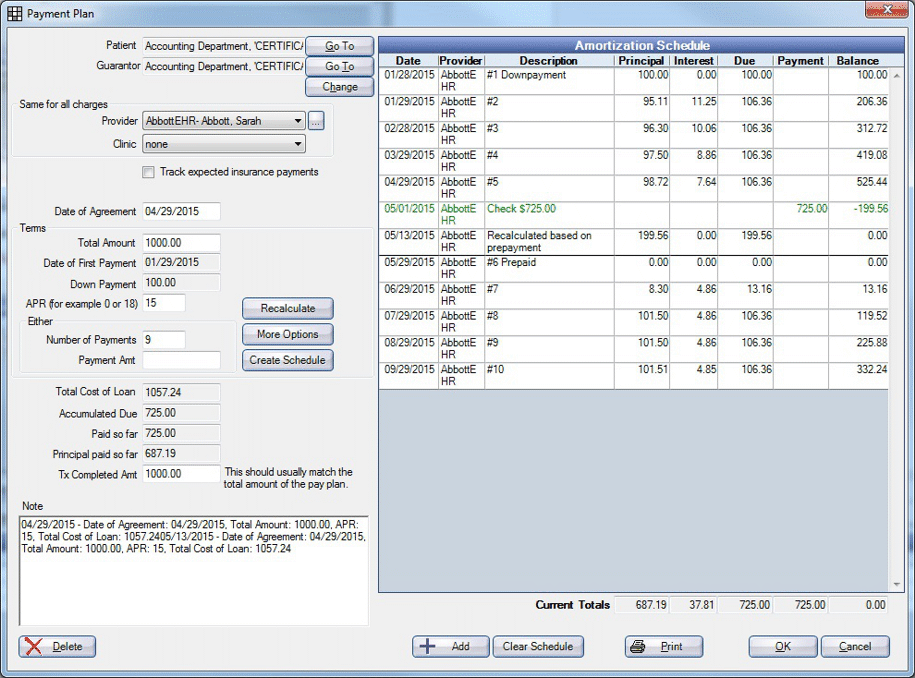2c. Overpaid: If you select Pay on Principal and Recalculate Interest2d. Overpaid: If you select Pay on Principal and do not Recalculate Interest.